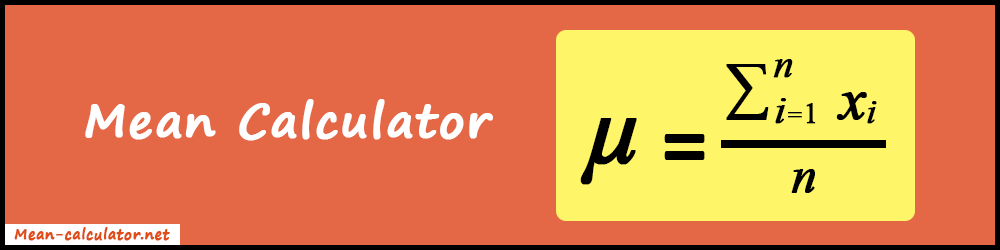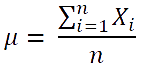# Mean Calculator

Mean Calculator helps to find the mean or average value from the given set of numbers. In addition, it gives median, mode, and range.

##### More Statistical Tools
Median Calculator Average Calculator Standard Normal Table

Mean Calculator is a free online tool for measuring the mean of a data set. Also, it's one of the best tools for doing calculations related to statistics and probability.

Find the mean for a sample set or population data within seconds. Also, it's loaded with a bunch of features that we will discuss further in the article.In statistics, we mainly use central tendency for representing values like mean, median, and mode to simply a large data set or population data. For determining the mean of a data set you can use our calculator.

Undoubtedly, it's one of the best tools you can find on the Internet for finding the mean value. Even more, it makes your work very easy and more accurate. No need to memorize the mathematical formula for calculating the mean anymore. Also, no need for pen and paper to do the manual calculations. Simply use our mean calculator and get your answer quickly.

Enter the input data set or paste it inside the input box and get your result instantly. Along with mean value, it also provides some additional useful results. Such as median, mode, range, geometric mean, root mean square, minimum and maximum value, count, and sum. Therefore, it's a very beneficial web application for students, teachers, researchers, and professionals.

In this article, we will know how to calculate mean with formula and example, its applications, key features, and how to use it. In short, we will discuss all the things regarding mean. So, stay with us and read the full article.

### What is Mean?

In simple terms, the mean is the average value of a set of numbers. Also, it is used to analyze the variability of data. In the context of statistics and probability, the mean represents the "Central Tendency" of a group of elements. Even more, it's one of the important statistical functions. It is beneficial for examining the common characteristics between the elements in a collection.

Mean, median, and mode these three terms are used for determining the "Variability" or "Central Tendency" of a data set containing numbers. But they have slight differences in how they individually work. Out of all, mean is the simplest.

#### Mean Formula:

In general, the mean is the sum of all the numbers in a set divided by the count of the set. Below is the mathematical representation for calculating the mean.In the above mathematical expression,

μ = mean

n = total number of elements in the data set= sum of all the elements (from the first element to the nth element)

### How to calculate Mean using Formula?

Let's take an example to understand the mean calculation. So, it can be easily grasped by beginners as well.

##### Example:

There has been a statistical survey and the values we got are as follows:
{7, 3, 1, 7, 4.6, 6.2, 10, 10.4, 7.5, 9.1}

##### Solution:

Step 1: Initialize the value of n
n = number of elements present in the set.
So, the total number of elements in the data set is 10.
Hence, the value of n = 10

Step 2: Find the sum of data set
Xi = 7 + 3 + 1 + 7 + 4.6 + 6.2 + 10 + 10.4 + 7.5 + 9.1 = 65.8

Step 3: Divide the sum of data set(Xi) by the total number of the elements(n)
μ = Xi / n = 65.8 / 10 = 6.58

Therefore, the mean (μ) = 6.58.

Under the hood of the mean calculator, the same algorithm is used for calculating the mean value. In short, it calculates the sum of all the values and then divides them by the count of the numbers. Along with it, it also performs certain other calculations. As a result, you can see many kinds of values in the result section.

#### Applications of Mean

Mean value is implemented by a bunch of mathematical, statistical, and probability equations. Here are some of the examples where the mean value of the operating data set is required:

• Standard deviation
• Standard error
• Poisson distribution
• T-distribution
• Variance
• Z-score
• Normal distribution
• Confidence interval
• Coefficient of variation
• Exponential distribution

Along with these, there can be thousand use cases apart from statistical work. Like for calculating the monthly average expenditure, the average speed of vehicles on road, productive hours in an office, etc...

#### Features of Mean Calculator

What makes our calculator stand as the best? Here are the points to answer this question.

• Only two-step process: To find the mean using our tool, it just takes two steps. Firstly, you need to enter the comma or space separated values. Secondly, just press the "Calculate" button. That's it for the work! Much better and convenient than pen-paper solving.
• Faster results: You get the result within a blink of a second.
• 100% Accurate: The calculation results are 100% accurate. There's no doubt about the result when a computer does the work. Provided that your input data set is correct according to you.
• Unlimited input values: There is no cap on the size of the input data set. So, you can have as many elements as you can.
• Easy to get started: No registration, login, or installation is needed for using the mean calculator. Just visit the website and get started quickly.
• Advanced results: Along with mean, you subsequently get values of Median, Mode, Range, Geometric Mean, Root Mean Square, Min. Value, Max. Value, Count, and Sum.

### How to use the Mean Calculator?

Now, it's time to know the steps required to use the mean calculator. Most importantly, you have to follow the proper steps in the order to get the desired result.

##### Pre-Requirements:

Whatever be your input data set, firstly, make sure that every element is a number. Whether it is an integer, decimal, fractional, or negative. Yes, our tool supports all types of values. So, you can input any type of number that is mentioned.

Then you need to separate them using the space or commas.

For example, if your input data set is from 11 to 15. So, you need to write: 11 12 13 14 15  or  11, 12, 13, 14, 15.

Using commas or spaces is mandatory, it helps the calculator to differentiate elements. Without separator, our calculator will not give you results.

1. Firstly, enter or paste the comma or space separated values into the input box. Trailing and leading whitespaces are ignored. So, no need to worry about them.
2. Press the "Calculate" button. As a result, you can see the mean and other statistical values for your data set just below.
3. For a new calculation just press the "Reset" button.

Yes, both terms are almost the same. It's a sum of all values divided by the number of elements.

Our tool is free to use an unlimited number of times. No hidden charges or anything. It's absolutely free.

Yes, you can find the mean of the data set having decimal, fractional and negative values.

Yes, our calculator works on all kinds of mobile devices. In addition, you can also use it on an iPad or laptop.

Unfortunately, our calculator doesn't support an infinite data set.

Enter all the values of your sample population separated by commas into the input field and press the "Calculate" button. As a result, you can see the geometric mean and root mean square for your data set in the result section.

No, it's not necessary to write values in ascending or descending order. You can enter the values in any way you like. Our calculator will automatically determine the order itself.